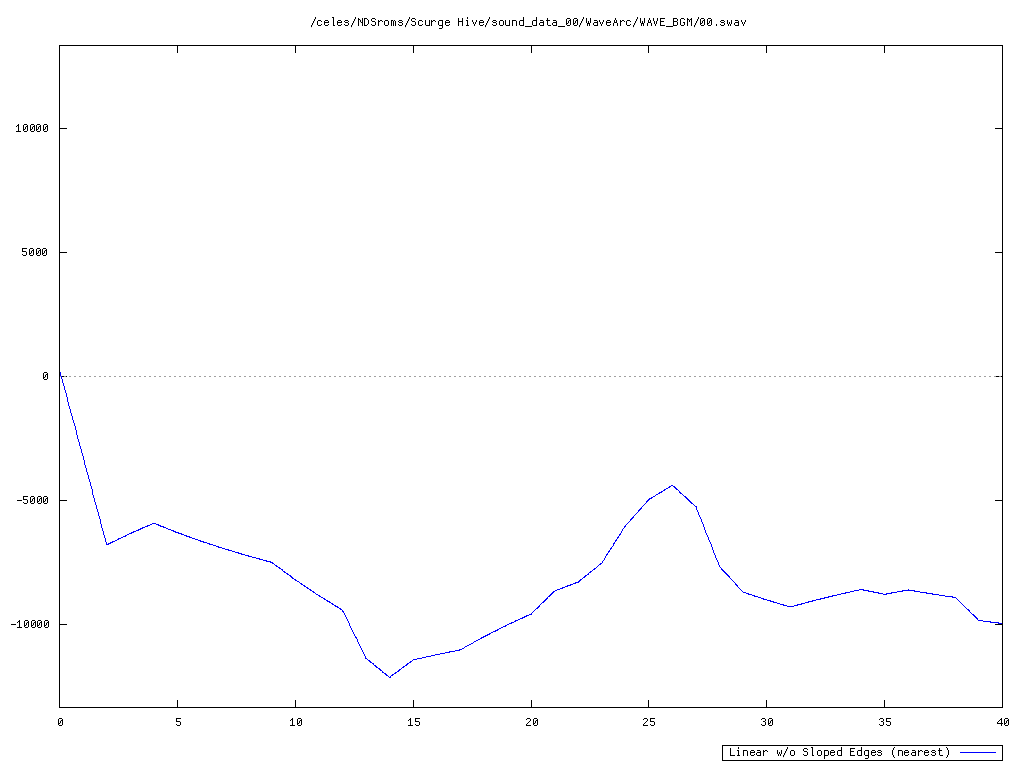Original SWAV (at 8927 Hz): Original.wav
Interpolated SWAV (at 44100 Hz): Linear without Sloped Edges (nearest)w/o Sloped Edges (nearest)
w/o Sloped Edges (current)
w/o Sloped Edges (zero)
w/ Sloped Edges

For data points that fall outside the range of the data (a.k.a. the edges of the data), the following occurs based on what is selected above:
• w/o Sloped Edges (nearest) = They will use the value of the data point directly next to them.
• w/o Sloped Edges (current) = They will use the value of the data point that was initially being referenced for the interpolation.
• w/o Sloped Edges (zero) = They will use zero for their value.
• w/ Sloped Edges = They will be calculated based on the slope of the data point directly next to them and the data point directly next to that one.
2-point, 3rd-order Optimal 2x
2-point, 3rd-order Optimal 4x
2-point, 3rd-order Optimal 8x
2-point, 3rd-order Optimal 16x
2-point, 3rd-order Optimal 32x
4-point, 2nd-order Optimal 2x
4-point, 2nd-order Optimal 4x
4-point, 2nd-order Optimal 8x
4-point, 2nd-order Optimal 16x
4-point, 2nd-order Optimal 32x
4-point, 2nd-order Parabolic 2x
4-point, 2nd-order Watte tri-linear
4-point, 3rd-order B-spline
4-point, 3rd-order Hermite
4-point, 3rd-order Lagrange
4-point, 3rd-order Optimal 2x
4-point, 3rd-order Optimal 4x
4-point, 3rd-order Optimal 8x
4-point, 3rd-order Optimal 16x
4-point, 3rd-order Optimal 32x
4-point, 4th-order Optimal 2x
4-point, 4th-order Optimal 4x
4-point, 4th-order Optimal 8x
4-point, 4th-order Optimal 16x
4-point, 4th-order Optimal 32x
4-point, 5th-order 2nd-order Osculating
6-point, 3rd-order Hermite
6-point, 4th-order Optimal 2x
6-point, 4th-order Optimal 4x
6-point, 4th-order Optimal 8x
6-point, 4th-order Optimal 16x
6-point, 4th-order Optimal 32x
6-point, 5th-order 2nd-order Osculating
6-point, 5th-order B-spline
6-point, 5th-order Hermite
6-point, 5th-order Lagrange
6-point, 5th-order Optimal 2x
6-point, 5th-order Optimal 4x
6-point, 5th-order Optimal 8x
6-point, 5th-order Optimal 16x
6-point, 5th-order Optimal 32x
Cosine
Linear
Sinc (Bartlett-Hann Window)
Sinc (Bartlett Window)
Sinc (Blackman-Harris Window)
Sinc (Blackman-Nuttall Window)
Sinc (Blackman Window)
Sinc (Cosine Window)
Sinc (Flat-Top Window)
Sinc (Guassian Window)
Sinc (Hamming Window)
Sinc (Hann-Poisson Window)
Sinc (Hann Window)
Sinc (Lanczos Window)
Sinc (Nuttall Window)
Sinc (Poisson Window)
Sinc (Rectangular Window)
Sinc (Welch Window)